# SAT Math Multiple Choice Question 707: Answer and Explanation

### Test Information

Question: 707

2.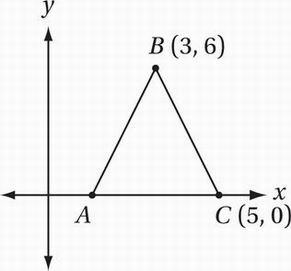Note: Figure not drawn to scale.

In the figure above, AB = BC. If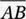has a slope of m and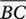has a slope of n, what is the value of mn?

• A. -9
• B.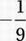• C.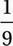• D. 9

If AB = BC, then triangle ABC is isosceles and therefore the two base angles are congruent and the triangle has a vertical axis of symmetry at the line x = 3. This implies that the slopes of linesandare opposites. We can calculate the slope offrom its endpoints: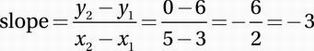Therefore, the slope ofis 3, and so mn = (3)(-3) = -9.